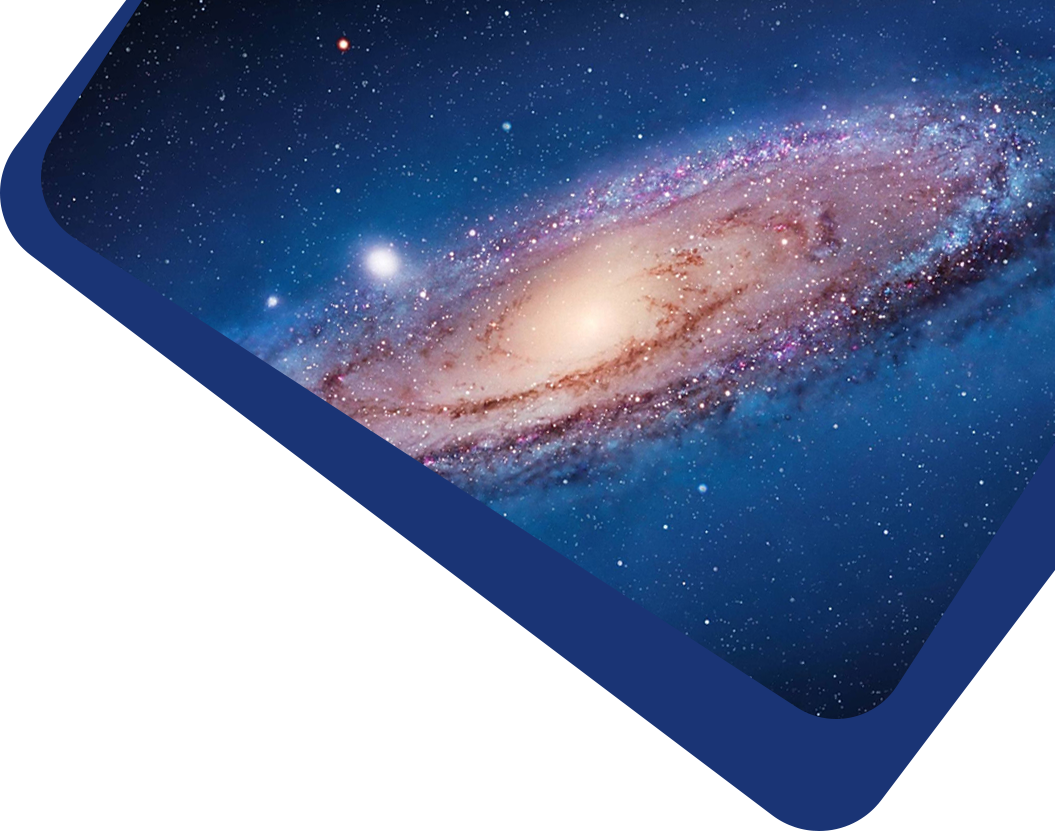Vol 22, No 7

## The Physical Properties and Starspot Activity of the Triple System KIC 6525196

#### Yang Pan, Jian-Ning Fu, Xiao-Bin Zhang, Jia-Xin Wang and Chun-Qian Li

Abstract

We present the results of photometric and spectroscopic analyses for the triple-lined system KIC 6525196, an eclipsing binary accompanied by a third star. By modeling the Kepler light curves and radial velocities from LAMOST and HIDES observations, absolute parameters of the system are determined. Both components of the eclipsing binary are found to be solar-like stars with masses and radii of $$M_1 = 1.0286 \pm 0.0026 M_{\odot}$$, $$R_1 = 1.127 \pm 0.008 R_{\odot}$$, and $$M_2 = 0.9667 \pm 0.0024 M_{\odot}$$, $$R_2 = 0.963 \pm 0.007 R_{\odot}$$. The mass of the third star is determined to be $$M_3 = 0.772 \pm 0.010 M_{\odot}$$. With the out-of-eclipse light residuals, we measure rotation period and decay timescale of an active region by using the autocorrelation function. In comparison to the Sun, the activity level of the system is significantly stronger. In addition, a possible short photometric activity cycle of 244 days is detected.

Keywords

stars: activity – (stars:) binaries: eclipsing – (stars:) binaries: spectroscopic

Full Text
Refbacks

• There are currently no refbacks.